Updating search results...

# 197 Results

View
Selected filters:
• fractions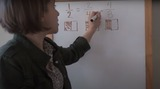Unrestricted Use
Public Domain
Rating
0.0 stars

This lesson is Day 2 in a series of 12 lessons around fraction equivalences and comparisons.

This video lesson uses paper folding to introduce the concept of equivalent fractions. To complete this lesson, make sure you have a square piece of paper (any size) and a crayon

Subject:
Mathematics
Material Type:
Activity/Lab
Interactive
Lesson
Simulation
Author:
Dawne Coker
06/25/2020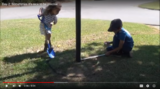Unrestricted Use
CC BY
Rating
0.0 stars

This lesson is Day 1 in a series of 5 lessons around U.S customary measurement.

This lesson focuses on measuring lengths to the nearest fourth of a inch, and discusses when it's necessary to find a precise measurement versus an estimate.

Subject:
Mathematics
Material Type:
Activity/Lab
Interactive
Lesson
Simulation
Author:
Dawne Coker
06/25/2020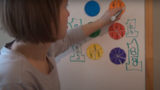Unrestricted Use
CC BY
Rating
0.0 stars

This lesson is Day 2 in a series of 12 lessons around fraction equivalences and comparisons.

This video lesson introduces the idea that fractions can be used to represent 1 whole. To engage in this lesson, students may use virtual circle models from Toy Theater (https://toytheater.com/fraction-circles/)

Subject:
Mathematics
Material Type:
Activity/Lab
Interactive
Lesson
Simulation
Author:
Dawne Coker
06/25/2020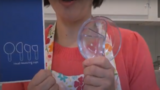Unrestricted Use
CC BY
Rating
0.0 stars

This lesson is Day 4 in a series of 12 lessons around fraction equivalences and comparisons.

This video lesson looks at how we use fractions in the kitchen. Students are challenged to use circle models to find equivalent fractions for a recipe. Students may use virtual circe models from the Toy Theater (https://toytheater.com/fraction-circles/).

Subject:
Mathematics
Material Type:
Activity/Lab
Interactive
Lesson
Simulation
Author:
Dawne Coker
06/25/2020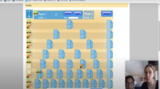Unrestricted Use
CC BY
Rating
0.0 stars

This lesson is Day 2 in a series of 12 lessons around fraction equivalences and comparisons.

This game may be played with one or two people. It reviews fractions on a number line, adding fraction with like denominators, and equivalent fractions. For an extra challenge, you can even break up your moves across multiple tracks (e.g., if your card reads 1/2, you could move 1/4 and 2/8).

https://www.nctm.org/Classroom-Resources/Illuminations/Interactives/Playing-Fraction-Track/

Subject:
Mathematics
Material Type:
Activity/Lab
Interactive
Lesson
Simulation
Author:
Dawne Coker
06/25/2020Unrestricted Use
CC BY
Rating
0.0 stars

This lesson is Day 6 in a series of 12 lessons around fraction equivalences and comparisons.

This video lesson teaches students how to create bar models to compare lengths/distances. Students will need a piece of paper, ruler, scissors, and a pencil. These bar models will also be used for Day 7's lesson.

Subject:
Mathematics
Material Type:
Activity/Lab
Interactive
Lesson
Simulation
Author:
Dawne Coker
06/25/2020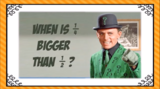Unrestricted Use
CC BY
Rating
0.0 stars

This video lesson introduces the idea that when comparing two fractions, they must refer to the same size whole. Then, students use fraction strips to compare fractions. Students may use virtual fraction strips from Toy Theater (https://toytheater.com/fraction-strips/)

Subject:
Mathematics
Material Type:
Activity/Lab
Interactive
Lesson
Simulation
Author:
Dawne Coker
06/25/2020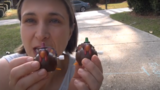Unrestricted Use
CC BY
Rating
0.0 stars

This lesson is Day 8 in a series of 12 lessons around fraction equivalences and comparisons.

This video lesson introduces the number as a tool for representing and comparing fractions. Students will need a black piece of a paper and a pencil with a good eraser.

Subject:
Mathematics
Material Type:
Activity/Lab
Interactive
Lesson
Simulation
Author:
Dawne Coker
06/25/2020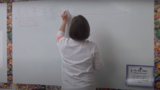Unrestricted Use
CC BY
Rating
0.0 stars

This lesson is Day 9 in a series of 12 lessons around fraction equivalences and comparisons.

This video lesson introduces students to fractions beyond 1 using a choral counting routine. Students connect the fractions to points on a number line and bar models. For this lesson, students need a piece of blank paper and a pencil.

Subject:
Mathematics
Material Type:
Activity/Lab
Interactive
Lesson
Simulation
Author:
Dawne Coker
06/25/2020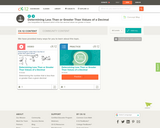Conditional Remix & Share Permitted
CC BY-NC-SA
Rating
0.0 stars

This short video and interactive assessment activity is designed to teach fifth graders about determining less than or greater than values of a decimal.

Subject:
Mathematics
Material Type:
Assessment
Interactive
Lecture
Provider:
CK-12 Foundation
Provider Set:
CK-12 Elementary Math
06/06/2019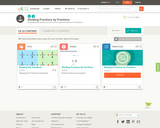Conditional Remix & Share Permitted
CC BY-NC-SA
Rating
0.0 stars

This short video and interactive assessment activity is designed to teach fifth graders about dividing fractions by fractions.

Subject:
Mathematics
Material Type:
Assessment
Interactive
Lecture
Provider:
CK-12 Foundation
Provider Set:
CK-12 Elementary Math
06/06/2019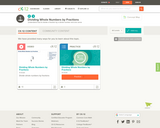Conditional Remix & Share Permitted
CC BY-NC-SA
Rating
0.0 stars

This short video and interactive assessment activity is designed to teach fifth graders about dividing whole numbers by fractions.

Subject:
Mathematics
Material Type:
Assessment
Interactive
Lecture
Provider:
CK-12 Foundation
Provider Set:
CK-12 Elementary Math
06/06/2019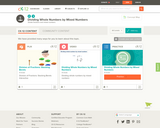Conditional Remix & Share Permitted
CC BY-NC-SA
Rating
0.0 stars

This short video and interactive assessment activity is designed to teach fifth graders about dividing whole numbers by mixed numbers.

Subject:
Mathematics
Material Type:
Assessment
Interactive
Lecture
Provider:
CK-12 Foundation
Provider Set:
CK-12 Elementary Math
06/06/2019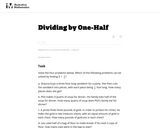Unrestricted Use
CC BY
Rating
0.0 stars

This task requires students to recognize both "number of groups unknown" and "group size unknown" division problems in the context of a whole number divided by a unit fraction.

Subject:
Mathematics
Material Type:
Activity/Lab
Provider:
Illustrative Mathematics
Provider Set:
Illustrative Mathematics
Author:
Illustrative Mathematics
05/01/2012Rating
0.0 stars

Students will use dominoes to learn how to represent a graphical model for adding unlike fractions.

Subject:
Mathematics
Material Type:
Activity/Lab
Lesson Plan
Provider:
PBS
Author:
Utah LessonPlans
02/26/2019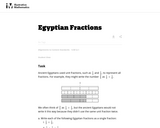Unrestricted Use
CC BY
Rating
0.0 stars

One goal of this task is to help students develop comfort and ease with adding fractions with unlike denominators. Another goal is to help them develop fraction number sense by having students decompose fractions. Because the Egyptians represented fractions differently than we do, it can also help students understand that there can be many ways of representing the same number.

Subject:
Mathematics
Material Type:
Activity/Lab
Provider:
Illustrative Mathematics
Provider Set:
Illustrative Mathematics
Author:
Illustrative Mathematics
08/09/2012Rating
0.0 stars

The math learning center is an app and online platform that allows students to use manipulatives virtually. In this activity, students will show equivalent fractions using lines and shapes.

Subject:
Applied Science
Computer Science
Material Type:
Activity/Lab
Game
Interactive
Module
Provider:
REMC Association of Michigan
Provider Set:
MiTechKids
Author:
REMC Association of Michigan
03/13/2019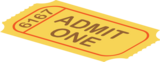Conditional Remix & Share Permitted
CC BY-NC-SA
Rating
0.0 stars

Exit Tickets for Grade 3 Fractions

Subject:
Mathematics
Material Type:
Formative Assessment
Author:
Drew Polly
07/12/2020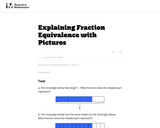Unrestricted Use
CC BY
Rating
0.0 stars

The purpose of this task is to provide students with an opportunity to explain fraction equivalence through visual models in a particular example.

Subject:
Mathematics
Material Type:
Activity/Lab
Provider:
Illustrative Mathematics
Provider Set:
Illustrative Mathematics
Author:
Illustrative Mathematics
06/25/2012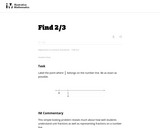Unrestricted Use
CC BY
Rating
0.0 stars

This simple-looking problem reveals much about how well students understand unit fractions as well as representing fractions on a number line.

Subject:
Mathematics
Material Type:
Activity/Lab
Provider:
Illustrative Mathematics
Provider Set:
Illustrative Mathematics
Author:
Illustrative Mathematics